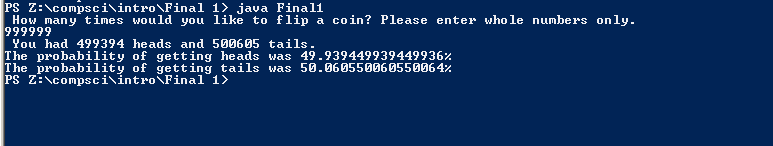# Fall Final, WE THE BEST!

## Code

```/// Name: Jonathan Stine
/// Period 5
/// Program Name: Coin Flip Final 1
/// File Name: Final1.java
/// Date Finished: 1/20/16

import java.util.Scanner;
import java.util.Random;

public class Final1
{

public static void main(String[] args)
{
Scanner input = new Scanner(System.in);

System.out.println( " How many times would you like to flip a coin? Please enter whole numbers only. " );
int n = input.nextInt();
while ( n < 1 || n > 2100000000 )
{

if ( n < 1 )
{
System.out.println("too small.");
}
else if ( n > 2100000000 )
{
System.out.println("too large.");
}

System.out.println("Please select a number between 1 and 2,100,000,000.");
n = input.nextInt();
}

int f = n;
/// This line here is used to "save" the ammount of flips in variable "f" before "n" gets altered in the coin flipper sequence.
int h = 0, t = 0;

Random r = new Random();
while ( n > 0 )
{
int x = r.nextInt(2);

if ( x == 0 )
{
h++;
n--;
} else if ( x == 1 )
{
t++;
n--;
}

}
double probHeads = 100 * (double)h / f;
double probTails = 100 * (double)t / f;
System.out.println(" You had " + h + " heads and " + t + " tails.");
System.out.println( "The probability of getting heads was " + probHeads + "%" );
System.out.println( "The probability of getting tails was " + probTails + "%" );

}
}

///High numbers divisible by 10 get the closest to a 50% to 50% ratio. Numbers like 1000 or 10000 work well to get as close to 50% as possible.

```

### Code Output